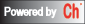## Model Conversion

A linear time-invariant (LTI) system can be modeled in three forms of representations: state-space equations, transfer function and zero-pole-gain representation. The following functions can be used to convert system model from one representation to another. The system before and after conversion are equivalent.

Note that the conversion from transfer function or zero-pole-gain representation to state-space equations are not unique.

Function
`    `
Description
To state-space model Find state-space equations for a system given transfer function or zero-pole-gain representation.
To transfer function model Find transfer function for a system given state-space equations or zero-pole-gain representation.
To ZPK model Find zero-pole-gain representation for a system given state-space equations or transfer function.